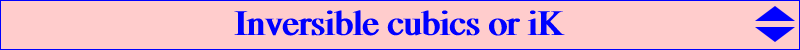An isocubic is said to be inversible when it is invariant under inversion with respect to the circumcircle of the reference triangle. It must be a pivotal isocubic denoted by iK(P) where P is the pivot of the cubic. P must be a point on the circumcircle. See Special isocubics §4.4 and CL032 for a generalization. See also Inverses of Isocubics. With P = (p:q:r), the general equation of such cubic is : ∑ qr x^2 (c^2 SC y - b^2 SB z) = 0. This cubic is always circular and is invariant under the isoconjugation which swaps P and O = X(3). Its pole lies on the circumconic with perspector X(184), the barycentric product of O and the circumcircle. All these cubics form a pencil containing : three degenerate cubics such that the line AO and the circle tangent at B, C to AB, AC, three focal cubics seen here, three symmetric (axial) cubics seen here. The tangents at A, B, C, P concur at O. The singular focus F is the Miquel point of the complete quadrilateral formed with the four tangents at A, B, C, P to the circumcircle. F lies on the circumcircle of the tangential triangle. Its inverse lies on the nine point circle. Such a cubic is always invariant (anallagmatic) under three other inversions whose (not all real) circles of inversion are orthogonal to the circumcircle and centered at the vertices of the diagonal triangle of the quadrilateral. These four circles are called focal circles and each one contains four of the sixteen (ordinary) foci of the cubic. Four of them are always real and lie on one of the four circles mentioned above. The isogonal transform of an inversible cubic is an antigonal cubic. See CL019. The following table shows a selection of such cubics (remember that the pole is the barycentric product of the pivot and O).pivot pole centers on the cubic cubic X(74) X(3), X(15), X(16), X(74) K114 X(98) X(248) X(3), X(98), X(485), X(486), X(1687), X(1688), X(2065) K336 X(99) X(3), X(99), X(249), X(525) X(100) X(906) X(3), X(59), X(100), X(521) X(103) X(3), X(103), X(916) X(104) X(1), X(3), X(36), X(90), X(104), X(912), X(1795) K436 X(107) X(112) X(3), X(107) see note (1) X(108) X(1415) X(3), X(108) X(110) X(3), X(110), X(250), X(520) X(111) X(3), X(6), X(111), X(187), X(895) X(112) X(1576) X(3), X(112) X(699) X(3), X(32), X(699), X(1691) X(741) X(3), X(58), X(295), X(741), X(1326) X(925) X(3), X(523), X(925) X(1113) X(3), X(1113), X(2574) X(1114) X(3), X(1114), X(2575) X(1141) X(3), X(13), X(14), X(54), X(96), X(265), X(539), X(1141), X(1157) K112 X(1290) X(3), X(1290), X(2850) X(1294) X(3), X(30), X(1294) X(1295) X(3), X(517), X(1295) X(1297) X(3), X(511), X(1297) X(1300) X(3), X(4), X(186), X(254), X(1300) X(1305) X(3), X(514), X(1305) X(2249) X(3), X(284), X(296), X(2249) X(2370) X(3), X(519), X(2370) X(2373) X(2), X(3), X(23), X(69), X(524), X(1177), X(2373) see note (2) K113 X(2374) X(3), X(25), X(468), X(2374) X(2694) X(3), X(2694), X(2771) X(2697) X(3), X(542), X(2697) X(2734) X(3), X(952), X(2734)(1) this is the only cubic of this type with pole on the circumcircle. (2) this is the only cubic of this type passing through its pole.